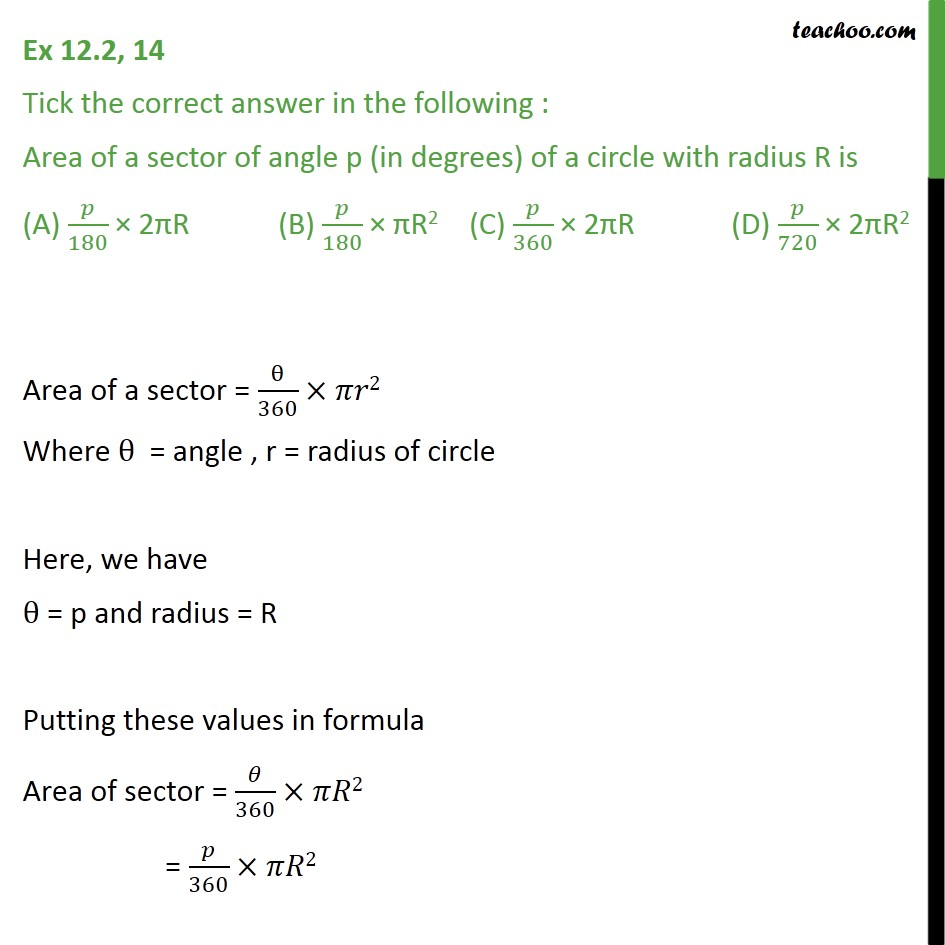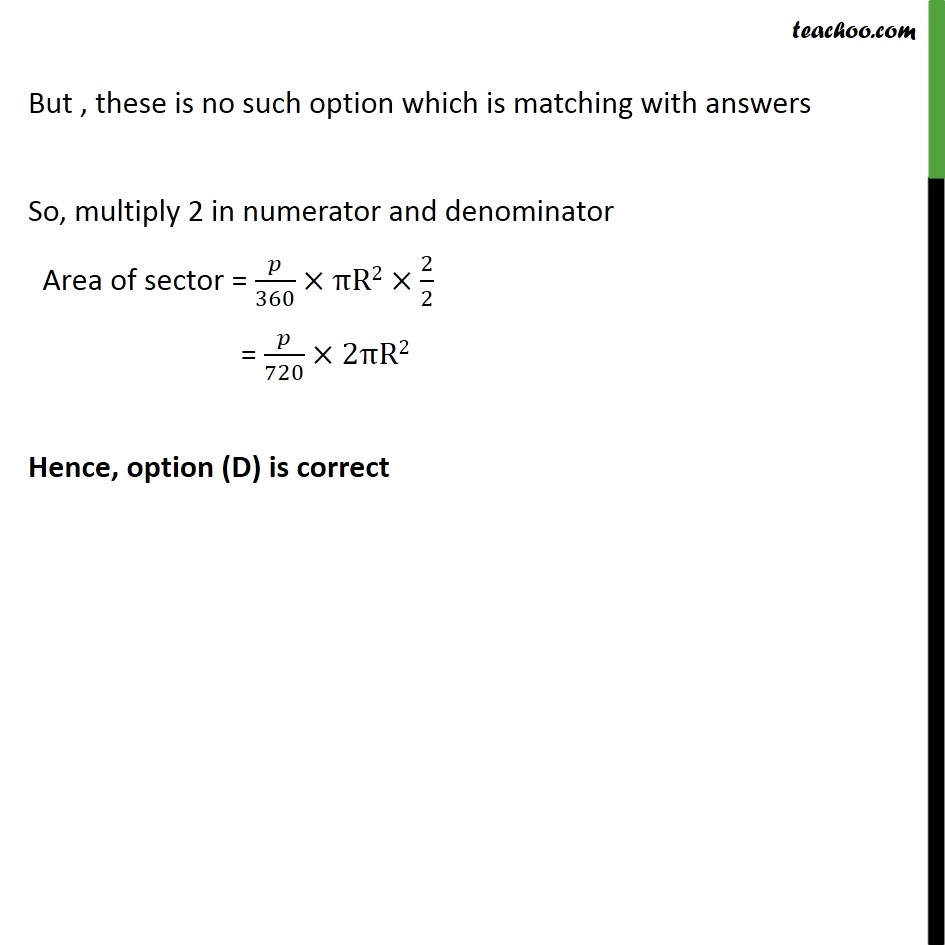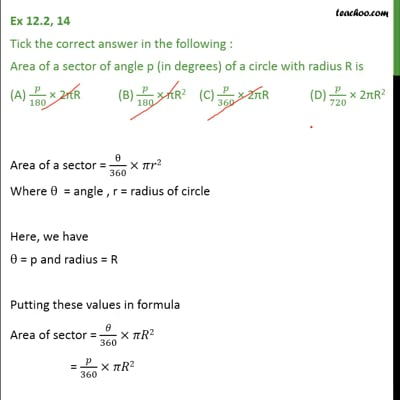Area of sector of circle

Chapter 12 Class 10 Areas related to Circles
Concept wiseThis video is only available for Teachoo black users

Introducing your new favourite teacher - Teachoo Black, at only ₹83 per month

### Transcript

Ex 12.2, 14 Tick the correct answer in the following : Area of a sector of angle p (in degrees) of a circle with radius R is (A) /180 2 R (B) /180 R2 (C) /360 2 R (D) /720 2 R2 Area of a sector = /360 2 Where = angle , r = radius of circle Here, we have = p and radius = R Putting these values in formula Area of sector = /360 2 = /360 2 But , these is no such option which is matching with answers So, multiply 2 in numerator and denominator Area of sector = /360 R2 2/2 = /720 2 R2 Hence, option (D) is correct